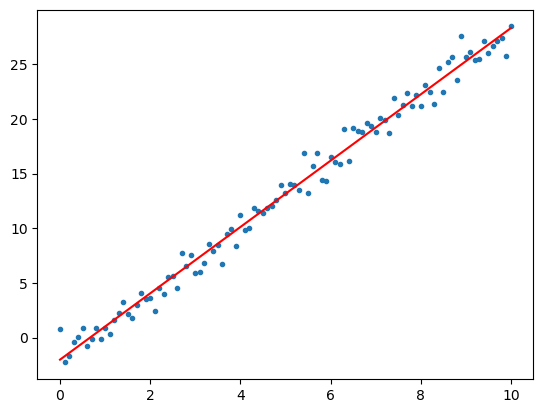# 9.3. Linear Systems and Regression¶

using LinearAlgebra


## 9.3.1. Linear Systems¶

One of the most common uses of matrices is for solving linear systems of equations. Julia uses the backslash operator \ for this:

A = [1 2; 3 4]
b = [5,1]
x = A \ b         # Solve Ax = b for x
A*x == b        # Confirm solution is correct

true


One way to view the syntax A\b is that it multiplies by A-inverse from the left, but using much more efficient and accurate algorithms.

For systems with many right-hand side vectors b, the \ operator also works with matrices:

B = [5 7; 1 -3]
X = A \ B          # Solve for two RHS vectors
A*X == B

false


The algorithm used by the \ operator is typically Gaussian elimination, but the details are quite complex depending on the type of matrices involved. Due to the high cost of general Gaussian elimination, it can make a big difference if you use a specialized matrix type:

n = 2000
T = SymTridiagonal(2ones(n), -ones(n))     # n-by-n symmetric tridiagonal

for rep = 1:3 @time T \ randn(n) end       # Very fast since T is a SymTridiagonal
Tfull = Matrix(T)                          # Convert T to a full 2D array
for rep = 1:3 @time Tfull \ randn(n) end   # Now \ is magnitudes slower

  0.203973 seconds (502.18 k allocations: 30.133 MiB, 25.28% gc time, 99.92% compilation time)
0.000047 seconds (4 allocations: 63.000 KiB)
0.000045 seconds (4 allocations: 63.000 KiB)
0.286723 seconds (368.03 k allocations: 51.592 MiB, 2.52% gc time, 51.66% compilation time)
0.120412 seconds (5 allocations: 30.564 MiB)
0.146118 seconds (5 allocations: 30.564 MiB, 17.15% gc time)


The matrix A in A\b can also be rectangular, in which case a minimum-norm least squares solution is computed.

## 9.3.2. Linear regression¶

Suppose you want to approximate a set of $$n$$ points $$(x_i,y_i)$$, $$i=1,\ldots,n$$, by a straight line. The least squares approximation $$y=a + bx$$ is given by the least-squares solution of the following over-determined system:

$\begin{split} \begin{pmatrix} 1 & x_1 \\ 1 & x_2 \\ \vdots & \vdots \\ 1 & x_n \end{pmatrix} \begin{pmatrix} a \\ b \end{pmatrix}= \begin{pmatrix} y_1 \\ y_2 \\ \vdots \\ y_n \end{pmatrix} \end{split}$
x = 0:0.1:10
n = length(x)
y = 3x .- 2 + randn(n)     # Example data: straight line with noise

A = [ones(n) x]            # LHS
ab = A \ y                 # Least-squares solution

using PyPlot
xplot = 0:10;
yplot = @. ab + ab * xplot
plot(x,y,".")
plot(xplot, yplot, "r");